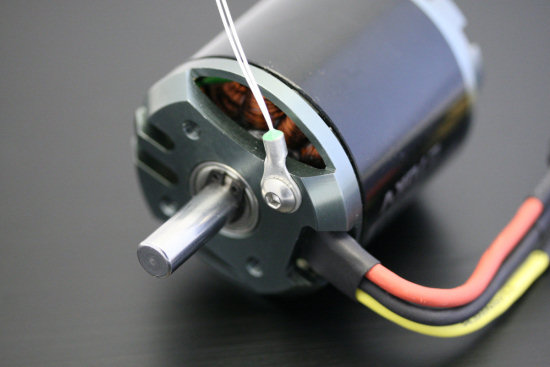How to measure a NTC thermistor via USB

# How to measure a NTC thermistor via USBYoctopuce provide three different methods to measure temperature, but there are even more techniques. Thermistors are another option: they are a family of electrical conductors whose resistance varies in a deterministic way based on their temperature, and that can therefore be used to measure temperature. So can we get anything out of them, for instance using a Yocto-Knob?

The two families of resistors varying according to temperature mainly used are:
- thermistors, in particular NTC (Negative Temperature Coefficient)
- platinium resistors, in particular the Pt100

Platinium resistors are very interesting because they are extremely precise. In fact, platinium resistors are used as reference to reproduce temperature scales internationally. But they are also quite expensive, and their use to get a precise measure requires more than a just bit of attention. We will have another opportunity to discuss them shortly.A thermistor can be used to measure the temperature on a specific point

NTC thermistors are also interesting, because they are a simple and inexpensive way of measuring the temperature at a specified point. For this kind of application, they are not as fast to react than thermocouples, and also only handle a limited temperature range (NTC usually cover -40°C to 125°C, while thermocouples can stand -200°C up to 1765°C). But NTC are much easier to measure than thermocouples. They can even be measured using a simple Yocto-Knob. Let’s see how.

## How to measure a resistance using a Yocto-Knob

The Yocto-Knob is a product designed to read up to 5 potentiometers, resistive sensors, phototransistors, switches or other similar sensors. It is an inexpensive device, without electrical isolation. With a simple software calibration, its internal software can provide a linear reading of a potentiometer, even when it does not match the input impedance. But it is not technically an Ohmmeter. The readings are intended to be used for interface knobs or event detection, not for calibrated measures.

It is nevertheless possible to estimate the value of the resistor connected to an input of the Yocto-Knob, using the get_rawValue() function. This function returns the raw 12bit value read on the analog input. It is therefore possible to use the following formula:Once the resistance is measured (or at least estimated), we can compute the temperature. It does not come out of a simple proportional rule, but there is a magic formula to get it, involving several parameters specific to each type of NTC :Some explanations :
- T is the temperature that we compute, expressed in Kelvins. To get it back to Celsius degrees, we will substract 273.
- R is the resistance measured on the NTC at a given time
- Ro and To define the reference point of the NTC, e.g. in our case 10KΩ at 25°C. The resistor must be specified in Ohms (hence 10,000 in our case) and the temperature in Kelvins (hence 273+25 in our case).
- B is the Beta factor specific to each NTC, and provided by the manufacturer. In our case, the Beta coefficient is 3976. The manufacturer names it Beta 25/85 to indicate that the parameter is only specified in the range between 25 and 85 °C. Outside this range, the formula might deviate from the actual resistance for this NTC.

This formula is easily translated into a computer program, for instance in Python:

def ntcTemp(R):
R0 = 10000
B = 3976
T0 = 273+25
return B / math.log( R / (R0*math.exp(-B/T0)) ) - 273

Now we are ready to write software to measure the temperature using a Yocto-Knob.A cost-effective solution to mesdure the temperature at a given point

## Software

We will take as starting point the Getting Started example from the Yocto-Knob, available in the Yoctopuce library. We just have to replace the main loop by the following code:

while True:
raw = channel1.get_rawValue()
line = 'Raw value: ' + str(raw)
if raw < 4092:
# a resistance is detected on the input
res = 12400 * raw / (4092 - raw) + 20
line += ' - resistance: ' + str(res)
if res > 340 and res < 340000:
line += ' - NTC temperature: ' + str(ntcTemp(res))
print(line)
YAPI.Sleep(500)

You can easily extend this code to handle the five inputs of the Yocto-Knob if you need to measure simultaneously the temperature at five different places. Since the price of a Yocto-Knob is approximately 34 USD, and that NTC price start under 3 USD, each measure channel comes out quite cheap. But for what kind of precision?

The resistance estimate is far from perfect, say 2% and 30Ω in the median range. In the median range, NTC resistance varies significantly, up to 450Ω per degree at 25°C. In the range 0°C-70°C, we can therefore estimate the precision of our measure at roughly +/- 1°C. A little experiment confirms these numbers:
- when dipped into cold water from melting ice blocks, the NTC reads as 32465Ω, or 0.9°C
- when hold at the surface of boiling water, the NTC reads 694Ω, or 99,47°C

Beyond this range, the uncertainty will rise, also due to the fact that the NTC formula may not stay exact outside of the specified Beta range. To fix this, you need to interpolate between fixed points specified in a resistance/tempereture table provided by the NTC manufacturer.

## And to get more precision ?

Now you have a cheap method to provide am estimate of the temperature and to detect significant changes, like something overheating. However, if you need to measure a temperature very precisely, like 0.03°C degrees, this would not fit the bill. But we are working on that as well, and a solution will come out shortly :-)

 Add a comment No comment yet Back to blog